# Another S-type Example b28c256

This chapter is an extension of the chapter we have titled, "Riemann Meets COLLADA & Oculus Rift S." In that chapter we used as our first example of a Riemann S-type ellipsoid the model with parameters, (b/a, c/a) = (0.41, 0.385). Here we construct a model with parameters, (b/a, c/a) = (0.28, 0.256). Other closely related chapters are listed below under the heading, "See Also".

## Key Physical Parameters

The model that we have chosen to use in our second successful construction of a COLLADA-based, 3D and interactive animation has the following properties; this model has been selected from Table 2 of our accompanying discussion of Riemann S-type ellipsoids: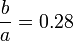$~\frac{b}{a} = 0.28$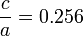$~\frac{c}{a} = 0.256$ Direct Adjoint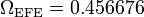$~\Omega_\mathrm{EFE} = 0.456676$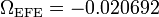$~\Omega_\mathrm{EFE} = - 0.020692$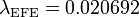$~\lambda_\mathrm{EFE} = 0.020692$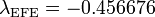$~\lambda_\mathrm{EFE} = - 0.456676$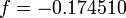$~f = - 0.174510$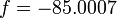$~f = - 85.0007$

The subscript "EFE" on Ω and λ means that the relevant frequency is given in units that have been adopted in [EFE], that is, in units of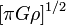$~[\pi G\rho]^{1 / 2}$. In Figure 1a, the yellow circular marker, that has been placed where the pair of purple dashed lines cross, identifies the location of this model in the "c/a versus b/a" EFE Diagram that appears as Figure 2 on p. 902 of S. Chandrasekhar (1965); essentially the same diagram appears in §49 (p. 147) of [EFE].

## Coding Steps

### Part A

Here we begin with a working model of b90c333 and use incremental changes in the COLLADA-based code to construct a working model of b28c256.

• This pair of starting models has been copied from the successful modeling of Riemann S-type ellipsoids that have (b/a, c/a) = (0.90, 0.333)
• Inertial Frame: [KEEP] FastInertial80.dae [04 June 2020]
• Rotating Frame: [KEEP] FastRot79.dae [04 June 2020]
• Pencil90.dae
Identical to FastRot79.dae except wall-mounted labeling has been changed to reflect new values of b/a and c/a. This works in both visualization venues.
• Pencil91.dae
Inserted correct surface geometry of this rapidly rotating Riemann ellipsoid. This works in both visualization venues.
• Pencil92.dae
Inserted the correct animated depiction of the 9 Lagrangian fluid elements.
• Pencil93.dae
Placed 51 yellow, equatorial-plane markers. This works in both visualization venues
• Pencil94.dae
Finished specifying correct behavior of clock, which gives the final, fully functional model. This works in both visualization venues
• PencilInertial95.dae
Flipped from rotating- to inertial-frame of reference. This works in both visualization venues
• PencilInertial96.dae
Enlarged red "Lagrange01" marker from 0.03 to 0.075; and changed transparency of ellipsoid surface to (totally opaque) 1.0. This works in both visualization venues

### Best b28c256 Models

The example models created for display in the Oculus Rift S are the following:

1. Inertial Frame: [KEEP] PencilInertial96.dae [04 June 2020]
2. Rotating Frame: [KEEP] Pencil94.dae [04 June 2020]

### Direct Configurations

b28c256DI.dae [Direct Inertial Frame]    …     a COLLADA code containing nnnn lines of <xml>-formatted ASCII text
Original filename used above: xxx.dae

<?xml version="1.0" encoding="UTF-8" standalone="no" ?>


b28c256DRot.dae [Direct Rotating Frame]    …     a COLLADA code containing nnnn lines of <xml>-formatted ASCII text
Original filename used above: xxx.dae

<?xml version="1.0" encoding="UTF-8" standalone="no" ?>